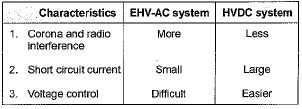Courses

# HVDC Of Power Systems

## 10 Questions MCQ Test Mock Test Series for Electrical Engineering (EE) GATE 2020 | HVDC Of Power Systems

Description
This mock test of HVDC Of Power Systems for Electrical Engineering (EE) helps you for every Electrical Engineering (EE) entrance exam. This contains 10 Multiple Choice Questions for Electrical Engineering (EE) HVDC Of Power Systems (mcq) to study with solutions a complete question bank. The solved questions answers in this HVDC Of Power Systems quiz give you a good mix of easy questions and tough questions. Electrical Engineering (EE) students definitely take this HVDC Of Power Systems exercise for a better result in the exam. You can find other HVDC Of Power Systems extra questions, long questions & short questions for Electrical Engineering (EE) on EduRev as well by searching above.
QUESTION: 1

Solution:
QUESTION: 2

### A dc line carries

Solution:

Due to requirement of less number of conductors, a dc line carries more power than a ac line, (losses of the line reduces in HVDC).

QUESTION: 3

### HVDC transmission is preferred to EHV AC because

Solution:

HVDC transmission is more reliable than EHV AC because it uses ground or sea return and therefore, in case of fault on a line it can still be used to supply power with the help of healthy lines.

QUESTION: 4

Back-to-back HVDC is used to

Solution:
QUESTION: 5

HVDC transmission needs

Solution:
QUESTION: 6

In HVDC system a converter acts as a rectifier when the firing angle α has a value

Solution:
QUESTION: 7

The capital cost on a HVDC converter is

Solution:
QUESTION: 8

In a dc transmision

Solution:

As operating frequency, f = 0 therefore, there is no effect of XL and XC.

QUESTION: 9

As compared to ac transmission dc transmission is free from

Solution:
QUESTION: 10

Which of the following is/are correctly matched?Solution: